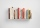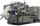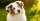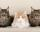The Hotel

The Holiday Hotel has the same number of rooms on each floor. Rooms are numbered with natural numbers sequentially from the first floor, no number is omitted, and each room has a different number. Three tourists arrived at the hotel. The first one was in room number 50 on the fourth floor. The other room number 100 on the seventh floor, third in room number 126 on the ninth floor. How many rooms are on each floor?

Result

n =  15

Solution:Leave us a comment of example and its solution (i.e. if it is still somewhat unclear...):Be the first to comment!To solve this example are needed these knowledge from mathematics:

Do you solve Diofant problems and looking for a calculator of Diofant integer equations?

Next similar examples:

1. TroopsRoute is long 147 km and the first day first regiment went at an average speed 12 km/h and journey back 21 km/h. The second day went second regiment same route at an average speed 22 km/h there and back. Which regiment will take route longer?
2. GardenArea of a square garden is 6/4 of triangle garden with sides 56 m, 35 m, and 35 m. How many meters of fencing need to fence a square garden?
3. PoolIf water flows into the pool by two inlets, fill the whole for 18 hours. The first inlet filled pool 6 hour longer than second. How long pool take to fill with two inlets separately?
4. Bookshelf and booksHow many can we place 7 books in a bookshelf?
5. Brick weightThe brick weighs 2 kg and a half bricks. How much does one brick weigh?
6. Forestry workersIn the forest is employed 56 laborers planting trees in nurseries. For 8 hour work day would end job in 37 days. After 16 days, 9 laborers go forth? How many days are needed to complete planting trees in nurseries by others, if they will work 10 hours a d
7. BonusGross wage was 527 EUR including 16% bonus. How many EUR were bonuses?
8. Motion problemFrom Levíc to Košíc go car at speed 81 km/h. From Košíc to Levíc go another car at speed 69 km/h. How many minutes before the meeting will be cars 27 km away?
9. Trapezoid MOThe rectangular trapezoid ABCD with right angle at point B, |AC| = 12, |CD| = 8, diagonals are perpendicular to each other. Calculate the perimeter and area of ​​the trapezoid.
10. Delayed clockMichael put a new battery into his watch at midnight. However, they are 5 seconds late each minute. How many hours does the watch show in 24 hours?
11. One threeWe throw two dice. What is the probability that max one three falls?
12. Assembly partsNine machines produce 1,800 parts on nine machines. How many hours will it produce 2 100 parts on seven such machines?
13. PupilsThere are 27 pupils in the classroom. They can swim 21 and ski nine pupils. Neither swim nor ski three pupils. How many pupils can swim and ski?
14. Dog priceTereza agreed to get a dog and 6000 crowns a year for help in a dachshund breeding station. After eight months she had to finish work and got a dog and 2000 crowns. What price does a dog have?
15. Probability of intersectionThree students have a probability of 0.7,0.5 and 0.4 to graduated from university respectively. What is the probability that at least one of them will be graduated?
16. Friction coefficientWhat is the weight of a car when it moves on a horizontal road at a speed of v = 50 km/h at engine power P = 7 kW? The friction coefficient is 0.07
17. Three catsIf three cats eat three mice in three minutes, after which time 260 cats eat 260 mice?